# Concept 3 – Scale Factor

Week 7 - Scale Factor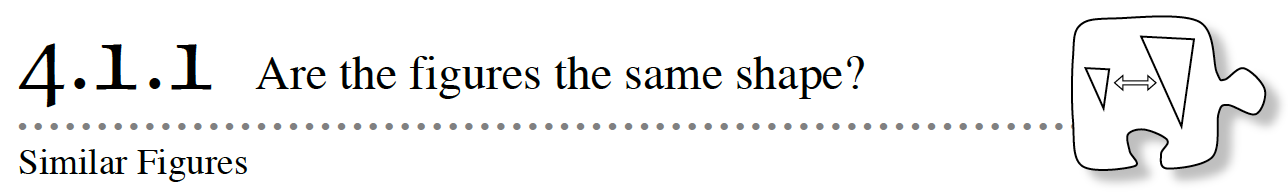Today you will extend your study of ratios by looking at enlargements and reductions of geometric figures.  Think of a copy machine and what it does to a picture when the “enlargement” button is selected.  The machine makes every length of the picture larger or smaller by multiplying it by the same number, called the multiplier.  That multiplier is also called the scale factor.

4-1. Karen is learning how to use the copy machine at her school’s main office and decides to scale the figure shown below by 300%.  She wonders what will happen to the figure.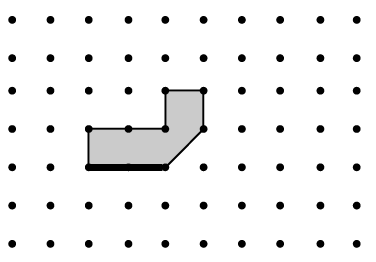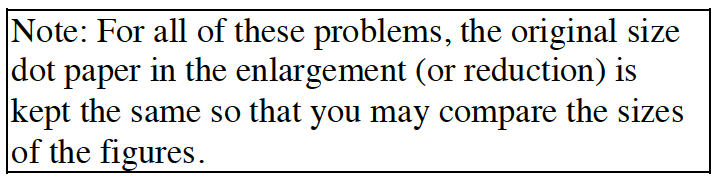a. Copy the original figure on your dot paper and label the length of each side.  Then scale the figure by 300%.  That is, make another copy of the figure and multiply each of the side lengths by 3.  Label the length of each new side.  What do you notice about the two figures?  Note the sides and the angles.

b.Refer to the darkened side on the original figure.  Then darken the corresponding (matchingside on the copy.  What is the length of this side on the original figure?  What is the length of this side on the copy?  Write and simplify the ratio of this pair of sides in the order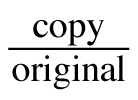c. Choose another pair of corresponding sides in the figures.  Write and simplify the ratio of these sides in the orderd. Predict the simplified ratio you would get for another pair of corresponding sides of the two figures.  Now test your prediction.  Write and simplify the ratio for the remaining pairs of corresponding sides.  Was your prediction correct?

e. Compare your simplified ratios from parts (b), (c), and (d).  What do you notice?  How do your answers relate to the scale factor of 300%?

4-2. Karen wants to try scaling the figure shown below by 50%.  What do you think will happen to the figure?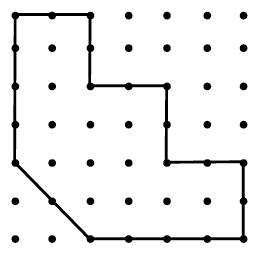a. Sketch the figure shown at right and make a copy of the figure scaled by 50% on your dot paper.  What is the same about the copy and the original?  What is different?

b. How is your copy different than the copy you made in problem 4-1?

c. Locate at least three pairs of corresponding sides.  There are nine in all.  Then write and simplify the ratio of each pair of corresponding sides in the orderd. Compare the ratios from each pair of corresponding sides with the scale factor.  What do you notice?  How do your ratios compare to the scale factor?

4-3. Examine the figure below.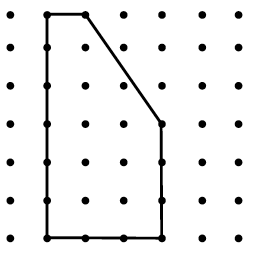a. Use dot paper to sketch the original figure.

b. With your team, choose a scale factor the will make the figure larger.  Sketch the copy.  What was your scale factor?  What is the ratio of the corresponding sides? How do the angles compare?

c. With your team, choose a scale factor that will make the figure smaller.  Sketch the copy.  What was your scale factor?  What is the ratio of the corresponding sides? How do the angles compare?

4-4. Similar figures are figures that have the same shape but are not necessarily the same size. One characteristic of similar shapes is that ratios of the sides of one figure to the corresponding sides of the other figure are all the same. Another characteristic is that the corresponding angles of the two figures are the same.

Patti claims she made a similar copy of each of the original figures shown in parts (a) and (b) below.  For each pair of figures, write and simplify the ratios for each pair of corresponding sides in the order.  Compare the ratios.  Are the figures similar?  That is, did Patti really make a copy?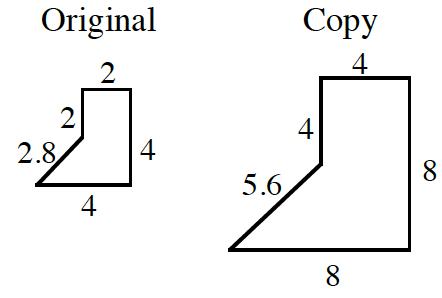Assignment: 4.1.1 Homework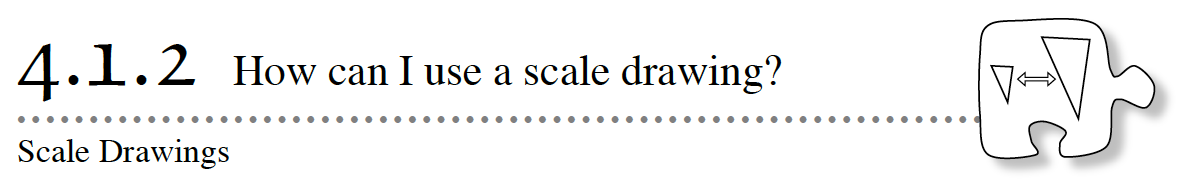Maps are examples of scale drawings.  They are reduced versions of the original regions.  A map is similar to the original region, because it has the same shape.  Because of this, maps conveniently allow users to determine distances between two points.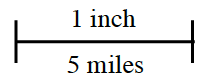In a scale drawing, it is important to decide on the unit of measure.  Maps made in the United States usually represent distances in miles, but they certainly cannot use actual miles as the unit of measure.  Otherwise, a map of Pennsylvania would be over 250 miles long and 450 miles wide!  A map includes a scale, which shows the units in which the map is drawn.  An example is shown at right.

4-12.  Guillermo needs a scale drawing of his house placed on its suburban lot.  The lot is 56′ wide and 100′ deep.  The garage is 20′ back from the street.  He has a sketch of his house – not drawn to scale – with the measurements shown below.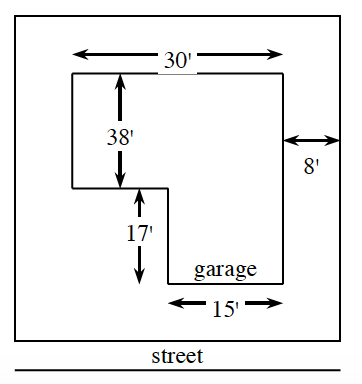a. Use graph paper and a ruler to create a scale drawing of Guillermo’s house on its lot.  Be sure to state your scale.

b. Guillermo wants to put a rectangular swimming pool in his backyard.  What is the largest pool you would advise him to have installed?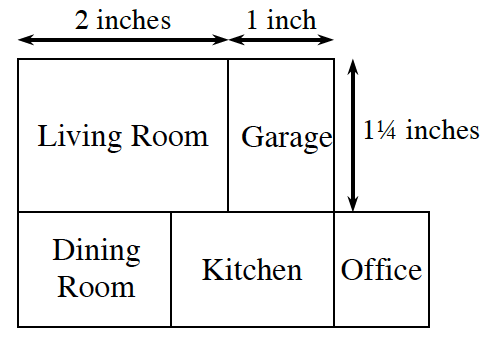4-13. The scale drawing at right shows the first floor of a house.  The actual dimensions of the garage are 20 feet by 25 feet.  All angles are right angles.

a. How many feet does each inch represent?  That is, what is the scale?

b. What are the length and width of the living room on the scale drawing (in inches)?

c. What are the actual length and width of the living room (in feet)?

d. If the family wants to lay carpet in the living room and carpeting costs \$1.25 per square foot, how much will the carpet cost?

4-15. Hank is planning his vegetable garden.  He has created the scale drawing below.  The actual area for the tomatoes will be 12 feet by 9 feet.  All angles are right angles.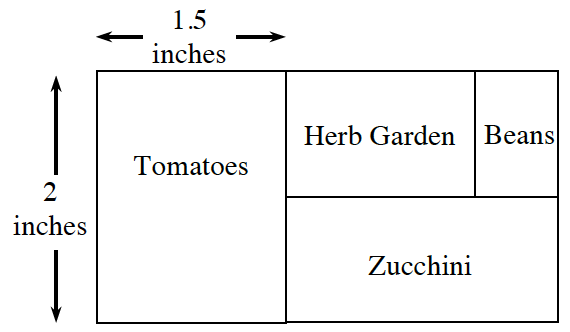a. What scale did Hank use?

b. If the horizontal length of the zucchini plot shown in the diagram is 1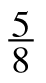, what is the area of the real vegetable garden in square feet?

Assignment: 4.1.2 Homework

School Logo Scaling

Lil Bot Big Bot and Mega Bot

Similar Figures – Kuta

Maya Floor Plan